## Series

### It's All Starting to Add Up

If sequences are the regular season, series are the Super Bowl. You need to be successful during the football season to get to the big game. Series build on sequences the same way. A series is nothing more than the sum of all the terms of a sequence. It just has some fancy-pants notation. If we were comparing math and baseball, series would be the World Series. (No relation.)Series will typically be written like so: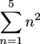Trust us when we say this looks way more intimidating than it actually is. The big guy there, ∑, is the Greek letter sigma. It means sum. This type of notation is technically called either sigma notation or summation notation. To-MAY-to, to-MAH-to.

The stuff to the right of the sigma (the n2 in this case) is the rule we're using to find the nth term.

The number written below the sigma tells you which term to start with. This is typically a 1 or a good ol' goose egg. The number up top will be where your terms finish. This top number can be whatever you'd like as well, but infinity is by far the greatest.

### Sample Problem

Find the sum: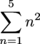.

All this problem is asking us to do is to add the sequence's terms up, starting with n = 1 (the first term) and ending with n = 5 (the fifth term). The explicit rule is {an} = n2. Listing the terms out we get:

a1 = 12

a2 = 22

a3 = 32

a4 = 42

a5 = 52

The giant sigma tells us to add those terms up. Here we go.

12 + 22 + 32 + 42 + 52 = Put that calculator away. What is this? Amateur hour?

1 + 4 + 9 + 16 + 25 = 55

So the sum of the first five terms is 55.

### Sample Problem

If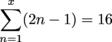, what's the value of x?

This is a little tougher, but we're just being asked to find the term number the series goes up to. The problem creator is likely just making sure we really understand how a series works.

All we need to do is start at n = 1 and add terms until we get to 16. Cake.

We'll start by adding the first two terms. Just plug in n = 1 and n = 2.

[2(1) – 1] + [2(2) – 1] = 1 + 3 = 4

Definitely not 16. Let's add the third term.

1 + 3 + [2(3) – 1] = 4 + 5 = 9

Warmer…

1 + 3 + 5 + [2(4) – 1] = 1 + 3 + 5 + 7 = 16

Bingo.

We added 4 terms, so x = 4.

### Sigmas, Constants, and You

Other neat-o math nerd tricks include multiplying sums by constants. Check it out: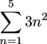is the same as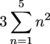and is equal to 3 × 55, which is otherwise known as 165. If there's a constant multiplied by the rule, we can totally slide it outside the sigma.

This is a basic concept that could get you out of a sticky situation or two. Like if a scary clown were ever to ask us to tell him what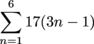was without a calculator, or else. We don't want to find out what "else" means.

### Sample Problem

You have been captured by a mathematically obsessed clown who would like to know the sum of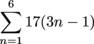. He makes a deal with you that if you tell him the answer without using a calculator, he'll let you go.

To start, we're going to use this constant multiple concept from before to rewrite the series.

That means that our series now looks like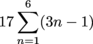This lets us deal with the sum first and the multiplication later.

Forget that pesky 17 for now and just find. Here goes…

[3(1) – 1] + [3(2) – 1] + [3(3) – 1] + [3(4) – 1] + [3(5) – 1] + [3(6) – 1] =

That step above is just the first term plus the second plus the third…up to six.

[3 – 1] + [6 – 1] + [9 – 1] + [12 – 1] + [15 – 1] + [18 – 1] =
2 + 5 + 8 + 11 + 14 + 17 =
57

Bringing 17 back into the sweet, sweet clown problem gives us…

17 × 57 = 969

See? Useful. We hate to say it, but we told you so.